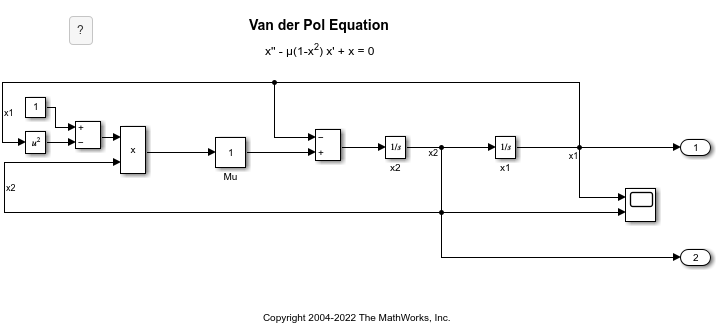# Collect Model Metrics Programmatically

You can use the model metric API to programmatically collect model metrics that help you assess the architecture, complexity, and readability of your model. The results of these metrics can help you verify compliance with industry standards and guidelines.

This example shows how to use the model metric API to programmatically collect subsystem and block count metrics for a model. After collecting metrics for the model, you can access the results and export them to a file.

### Example Model

Open the `vdp` model.

```model = 'vdp'; open_system(model);```### Collect Metrics

To collect metric data on a model, create an `slmetric.Engine` object and call `execute`.

`metric_engine = slmetric.Engine();`
```Warning: The Metrics Dashboard and slmetric.Engine API will be removed in a future release. For size, architecture, and complexity metrics, use the Model Maintainability Dashboard and metric.Engine API instead. The Model Maintainability Dashboard and metric.Engine API can identify outdated metric results, analyze dependencies between files, and aggregate metrics across software units and components. For more information, see <a href="matlab:helpview([docroot '/slcheck/collect-model-metric-data-1.html'])">Collect Model and Testing Metrics</a> ```
```setAnalysisRoot(metric_engine,'Root','vdp','RootType','Model'); execute(metric_engine);```
```Updating Model Advisor cache... Model Advisor cache updated. For new customizations, to update the cache, use the Advisor.Manager.refresh_customizations method. ```

### Access Results

Use the `getMetrics` method to specify the metrics you want to collect. For this example, specify the block count and subsystem count metrics for the `vdp` model. `getMetrics` returns an array of `slmetric.metric.ResultCollection` objects.

```res_col = getMetrics(metric_engine,{'mathworks.metrics.SimulinkBlockCount',... 'mathworks.metrics.SubSystemCount'});```

### Store and Display Results

Create a cell array called `metricData` to store the `MetricID`, `ComponentPath`, and `Value` properties for the metric results. The `MetricID` property is the identifier for the metric, the `ComponentPath` property is the path to component for which the metric is calculated, and the `Value` property is the metric value. Write a loop to display the results.

```metricData ={'MetricID','ComponentPath','Value'}; cnt = 1; for n=1:length(res_col) if res_col(n).Status == 0 results = res_col(n).Results; for m=1:length(results) disp(['MetricID: ',results(m).MetricID]); disp([' ComponentPath: ',results(m).ComponentPath]); disp([' Value: ',num2str(results(m).Value)]); metricData{cnt+1,1} = results(m).MetricID; metricData{cnt+1,2} = results(m).ComponentPath; metricData{cnt+1,3} = results(m).Value; cnt = cnt + 1; end else disp(['No results for:',res_col(n).MetricID]); end disp(' '); end```
```MetricID: mathworks.metrics.SimulinkBlockCount ```
``` ComponentPath: vdp ```
``` Value: 13 ```
```MetricID: mathworks.metrics.SimulinkBlockCount ```
``` ComponentPath: vdp/More Info ```
``` Value: 1 ```
```MetricID: mathworks.metrics.SimulinkBlockCount ```
``` ComponentPath: vdp/More Info/Model Info ```
``` Value: 1 ```
```MetricID: mathworks.metrics.SimulinkBlockCount ```
``` ComponentPath: vdp/More Info/Model Info/EmptySubsystem ```
``` Value: 0 ```
``` ```
```MetricID: mathworks.metrics.SubSystemCount ```
``` ComponentPath: vdp ```
``` Value: 1 ```
```MetricID: mathworks.metrics.SubSystemCount ```
``` ComponentPath: vdp/More Info ```
``` Value: 0 ```
```MetricID: mathworks.metrics.SubSystemCount ```
``` ComponentPath: vdp/More Info/Model Info ```
``` Value: 1 ```
```MetricID: mathworks.metrics.SubSystemCount ```
``` ComponentPath: vdp/More Info/Model Info/EmptySubsystem ```
``` Value: 0 ```
``` ```

### Export Results

To export the `MetricID`, `ComponentPath`, and `Value` to a spreadsheet, use `writetable` to write the contents of `metricData` to `MySpreadsheet.xlsx`.

```filename = 'MySpreadsheet.xlsx'; T=table(metricData); writetable(T,filename);```

To export the metric results to an XML file, use the `exportMetrics` method. For each metric result, the XML file includes the `ComponentID`, `ComponentPath`, `MetricID`, `Value`, `AggregatedValue`, and `Measure`.

```filename='MyMetricResults.xml'; exportMetrics(metric_engine,filename)```

Close the `vdp` model.

`bdclose(model);`

### Limitations

When you collect metric data, it is stored in a database file, `Metrics.db`, inside the simulation cache folder. You cannot collect metric data on one platform, move the database file to another platform, and then continue to collect additional metric data in that database file. For example, if you collect metric data on a Windows machine and then move the database file to a Linux machine, you cannot collect and store additional metric data in that database file. However, you are able to view that data in the Metrics Dashboard.